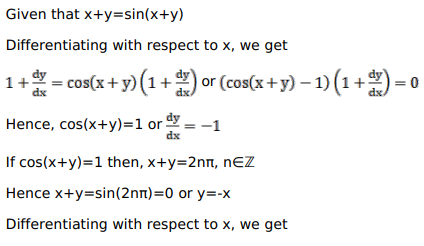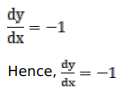# Solve this following

Question:

Mark $(\sqrt{ })$ against the correct answer in the following:

If $(x+y)=\sin (x+y)$ then $\frac{d y}{d x}=?$

A. -1

B. 1

C. $\frac{1-\cos (x+y)}{\cos ^{2}(x+y)}$

D. none of these

Solution: